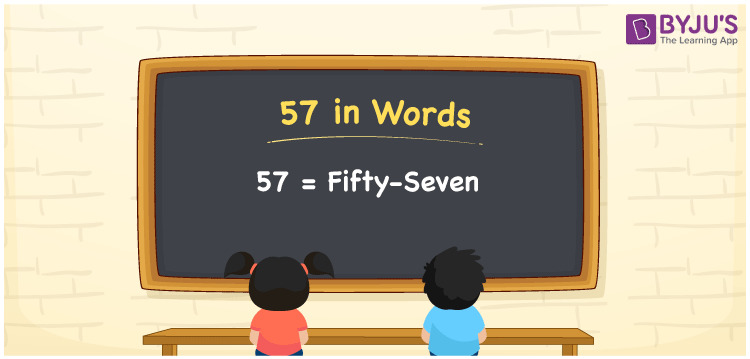# 57 in words

57 in words is written as Fifty Seven. 57 represents the count or value. The article on Counting Numbers can give you an idea about count or counting. The number 57 has four factors. They are 1, 3, 19 and 57. It is used at multiple places and instances. For example, “This garment factory was established 57 (Fifty Seven) years ago.” Another example: “A Yoga center can have Fifty Seven people practicing at the same time.”

 57 in words Fifty Seven Fifty Seven in Numbers 57

## 57 in English Words## How to Write 57 in Words?

We can convert 57 to words using a place value chart. The number 57 has 2 digits. Let us check how 57 would look like in a chart that shows the place value up to 2 digits.

 Tens Ones 5 7

Thus, we can write the expanded form as:

5 × Ten + 7 × One

= 5 × 10 + 7 × 1

= 57

= Fifty Seven.

57 is thenatural numberthat is succeeded by 56 and preceded by 58.

57 in words – Fifty Seven.

Is 57 an odd number? – Yes.

Is 57 an even number? – No.

Is 57 a perfect square number? – No.

Is 57 a perfect cube number? – No.

Is 57 a prime number? – No

Is 57 a composite number? – Yes

## Solved Example

1. Write the number 57 in expanded form

Solution: 5 x 10 + 7 x 1

We can write 57 = 50 + 7

= 5 x 10 + 7 x 1

## Frequently Asked Questions on 57 in words

### How to write 57 in words?

57 in words is written as Fifty Seven.

### State if True or False. 57 is an even number and hence it is divisible by 2?

False. 57 is an odd number and it is not divisible by 2.

### Can we express 57 as a product of two numbers?

Yes. 57 can be expressed as 1 × 57 or 3 × 19